# KSEEB SSLC Class 10 Maths Solutions Chapter 10 Quadratic Equations Ex 10.3

KSEEB SSLC Class 10 Maths Solutions Chapter 10 Quadratic Equations Ex 10.3 are part of KSEEB SSLC Class 10 Maths Solutions. Here we have given Karnataka SSLC Class 10 Maths Solutions Chapter 10 Quadratic Equations Exercise 10.3.

## Karnataka SSLC Class 10 Maths Solutions Chapter 10 Quadratic Equations Exercise 10.3

Question 1.
Find the roots of the following quadratic equations, if they exist, by the method of completing the square:
(i) 2x2 – 7x + 3 = 0.
(ii) 2x2 + x – 4 = 0
(iii) 4x2 + $$4 \sqrt{3} x$$ + 3 = 0
(iv) 2x2 + x + 4 = 0
Solution:
(i) 2x2 – 7x + 3 = 0
Dividing all terms by 2,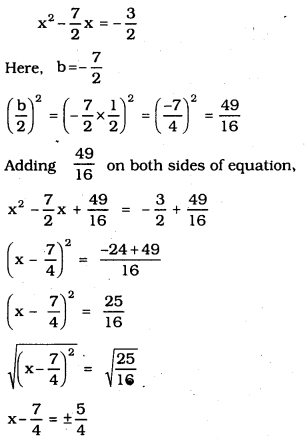(ii) 2x2 + x – 4 = 0
Dividing all terms by 2,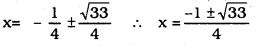(iii) 4x2 + $$4 \sqrt{3} x$$ + 3 = 0
Dividing all terms by 4,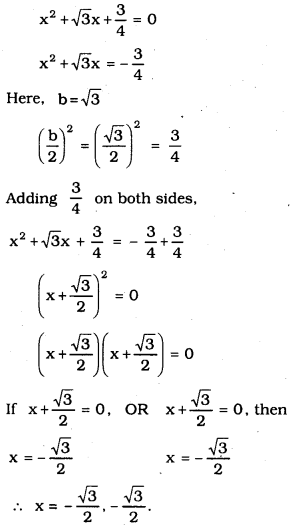(iv) 2x2 + x + 4 = 0
Dividing all terms of the equation by 2,Here ‘x’ has no fixed value because $$\sqrt{-31}$$ is not a square root.

Question 2.
Find the roots of the quadratic equations given in Q.1 above by applying the quadratic formula.
(i) 2x2 – 7x + 3 = 0.
(ii) 2x2 + x – 4 = 0
(iii) 4x2 + $$4 \sqrt{3} x$$ + 3 = 0
(iv) 2x2 + x + 4 = 0
Solution:
(i) 2x2 – 7x + 3 = 0.
Here, a = 2, b = -7, c = 3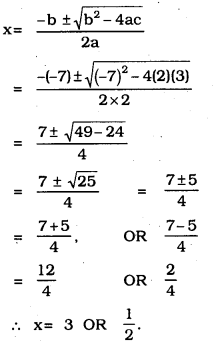(ii) 2x2 + x – 4 = 0
Here, a = 2, b = 1, c = 4(iii) 4x2 + $$4 \sqrt{3} x$$ + 3 = 0
Here, a = 4, b = $$4 \sqrt{3}$$, c = 3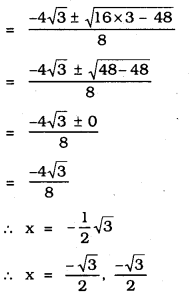(iv) 2x2 + x + 4 = 0
Here, a = 2, b = 1, c = 4Question 3.
Find the roots of the following equations: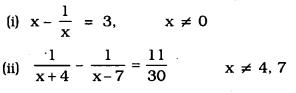Solution: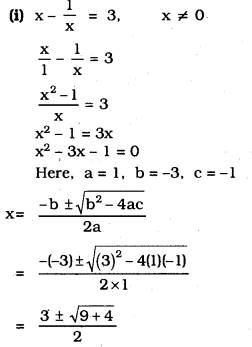(x – 2) (x – 1) = 0
If x – 2 = 0, then x = 2
If x – 1 = 0, then x = 1
∴ x = 2 OR x = 1.

Question 4.
The sum of the reciprocals of Rehman’s ages, (in years) 3 years ago and 5 years from now is $$\frac{1}{3}$$. Find his present age.
Solution:
Let the present age of Rehman be ‘x’ years.
After 5 years his age will be (x + 5)
3 years back his age was (x – 3)(x – 7) (x + 3) = 0
If x – 7 = 0, then x = 7
If x + 3 = 0, then x = -3
∴ x = 7 OR x = -3
∴ Present age of Rehman is 7 years.

Question 5.
In a class test, the sum of Shefali’s marks in Mathematics and English is 30. Had she got 2 marks more in Mathematics and 3 marks less in English, the product of their marks would have been 210. Find her marks in the two subjects.
Solution:
Let Shefali’s marks in mathematics is Y and marks in English is (30 – x)
Shefali’s gets 2 more marks in mathematics then x + 2 and 3 marks less in English is (30 – x – 3)
Product of Shefali’s marks = 210
(x + 2) (30 – x – 3) = 210
(x + 2) (27 – x) = 210
27x + 54 – x2 – 2x = 210
– x2 + 25x + 54 = 210
x2 – 25x + 210 – 54 = 0
x2 – 25x + 156 = 0
x2 – 13x – 12x + 156 = 0
x(x – 13) – 12 (x – 13) = 0
(x – 13) (x – 12) = 0
x – 13 = 0 (or) x – 12 = 0
x = 13 (or) x = 12
Therefore, Shefali’s marks in Mathematics =13
Marks in English = 30 – 13 = 17 (or) Shefali’s marks in Mathematics = 12 then.
Marks in English = 30 – 12 = 18

Question 6.
The diagonal of a rectangular field is 60 metres more than the shorter side. If the longer side is 30 metres more than the shorter side, find the sides of the field.
Solution: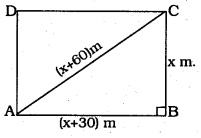Let shorter side of rectangle ABCD be x metre,
Then, longer side of rectangle ABCD,
AB = (x + 30) m.
Diagonal AC = (x + 60) m.
In ⊥∆ABC, ∠B = 90°.
As per Pythagoras theorem,
AB2 + BC2 = AC2
(x + 30)2 + (x)2 = (x + 60)2
x2 + 60x + 900 + x2 = x2 + 120x + 3600
2x2 + 60x + 900 = x2 + 120x + 3600
2x2 – x2 + 60x – 120x + 900 – 3600 = 0
x2 – 60x – 2700 = 0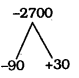x2 – 90x + 30x – 2700 = 0
x(x – 90) + 30 (x – 90) = 0
(x – 90) (x + 30) = 0
If x – 90 = 0, then x = 90
If x + 30 = 0, then x = -30
Shorter side of rectangle, BC = x = 90 m.
Longer side of rectangle, AB = x + 30 = 90 + 30= 120 m.
Diagonal of rectangle, AC = x + 60 = 90 + 60 = 150 m.

Question 7.
The difference of squares of two numbers is 180. The square of the smellier number is 8 times the larger number. Find the two numbers.
Solution:
Let the small number be ‘x’.
The Square of the smaller number is 8 times the larger number.
∴ x2 = 8 × Larger number.
∴ Larger number = $$\frac{1}{8}$$ x2.
The difference between these numbers is 180.∴ Smaller number is 12,
Larger number is 18.

Question 8.
A train travels 360 km at a uniform speed. If the speed had been 5 km/h more, it would have taken 1 hour less for the same journey. Find the speed of the train.
Solution:
Let initial speed of the train be x km/h,
Then, time taken to cover 360 km
If the speed had been 5 km/h more,
$$\frac{360}{x}$$ Hr.
then time taken to cover is $$\frac{360}{x+5}$$ Hr.x(x + 365) = 360 (x + 5)
x2 + 365x = 360x + 1800
x2 + 365x – 360x – 1800 = 0
x2 + 5x – 1800 = 0
x2 + 45x – 40x – 1800 = 0
x(x + 45) – 40 (x + 45) = 0
(x + 45) (x – 40) = 0
If x + 45 = 0, then x = – 45
If x – 40 = 0, then x = 40
∴ Initial speed of a train is 40 km/hr.

Question 9.
Two water taps together can fill a tank in $$9 \frac{3}{8}$$ hours. The tap of a larger diameter takes 10 hours less than the smaller one to fill the tank separately. Find the time in which each tap can separately fill the tank. .
Solution:
Let the time required to fill up the tank for tap having a larger diameter be ‘x’ Hr. For ‘x’ hour part of the tank filling is 1.
For $$9 \frac{3}{8}$$ hr. part of the tank filling ………..?
i.e., $$\frac{75 \times 1}{8 x}$$ part
Time required for smaller diameter is = (x – 10)Hr.
For (x – 10)Hr part of the tank, filling is 1
For $$9 \frac{3}{8}$$ Hr. part of the tank is …………?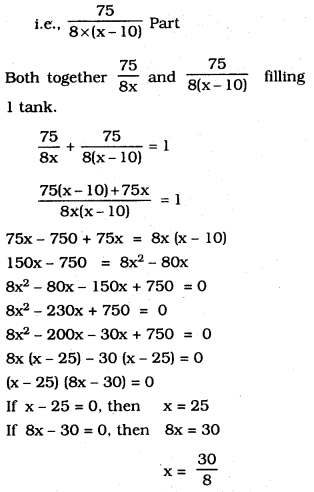∴Time required for larger diameter, x = 25 Hr.
∴ Time required for smaller diameter = x – 10 = 15 Hr.

Question 10.
An express train takes 1 hour less than a passenger train to travel 132 km between Mysore and Bangalore (without taking into consideration the time they stop at intermediate stations). If the average speed of the express train is 11km/h more than that of the passenger train, find the average speed of the two trains.
Solution:
Let Average speed of passenger train be = x km/h.
. But Average speed of Express train be = (x + 11) km/h.
Time required for Passenger:
For travelling x km time required is 1 Hr
For travelling 132 km time required …….?
$$\frac{132}{x}$$ Hr.
Time required for Express train :
For travelling (x + 11) km is 1 Hr.
For travelling 132 km …………?
i.e., $$\frac{132}{(x+11)}$$ Hr
Express train required 1 Hr. less comparing to Passenger train. .x(x + 143) = 132 (x+ 11)
x2 + 143x = 132x + 1452
x2 + 143x – 132 x – 1452 = 0
x2 + 11x – 1452 = 0
x2 + 44x – 33x – 1452 = 0
x(x + 44) – 33(x + 44) = 0
(x + 44) (x – 33) = 0
If x + 44 = 0, then x = -44
If x – 33 = 0, then x = 33
∴ Average speed of passenger train is 33 km/hr.
∴ Average speed of Express train = x + 11 = 33 + 11 = 44 km/hr.

We hope the given KSEEB SSLC Class 10 Maths Solutions Chapter 10 Quadratic Equations Ex 10.3 will help you. If you have any query regarding Karnataka SSLC Class 10 Maths Solutions Chapter 10 Quadratic Equations Exercise 10.3, drop a comment below and we will get back to you at the earliest.

error: Content is protected !!